# Electronics and Communication Engineering - Exam Questions Papers

11.

Find the voltage across 64 Ω resistor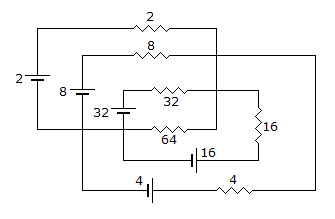A.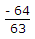V B.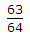V C.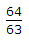V D.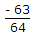V

Explanation: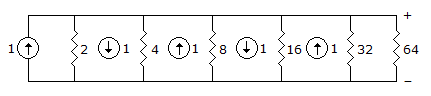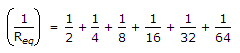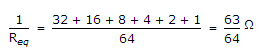Voltage across 64 Ω is = 1x=V.

12.

If f(Z) = c0 + c1z-1, then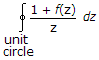is given by

 A. 2pc1 B. 2p (1 + c0) C. 2pjc1 D. 2pj(1 + c0)

Explanation: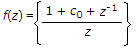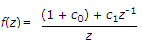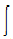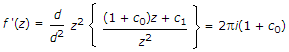13.

The capacitors C1, C3, C4 and C5 have a capacitance of 4 μF each and the capacitor C2 has a capacitance of 10 μF. The effective capacitance (in μF) between the points X and Y will be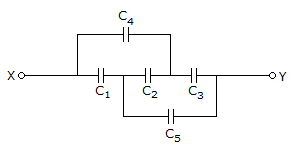A. 4 μF B. 6 μF C. 3 μF D. 8 μF

Explanation: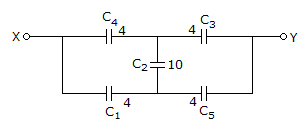Converting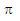into T network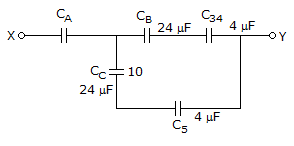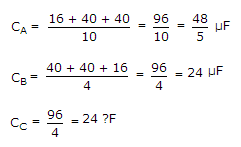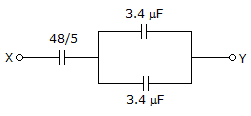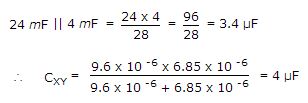14.

(9)11 x (9)13 x(9)15 = (?)16

 A. 933 B. 2D9 C. 339 D. 9AB

Explanation:

(9 x 9 x 9)15 = (729)15 = (2D9)16 .

15.

The equivalent T circuit of this coupled network is shown in figure. Find the values of ZA, ZB and ZC.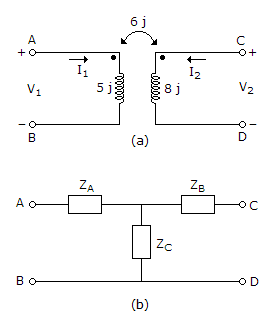A. -j, 6j, 2j B. -j, 2j, 6j C. j, 2j, - 6j D. j, 2j, 6j

Explanation:

Use mesh analysis for the coupled network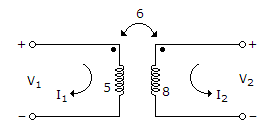Apply KVL at loop 1

V1 = 5jI1 + 6jI2

Apply KVL to loop 2

V2 = 8jI2 + 6jI1

Consider given T network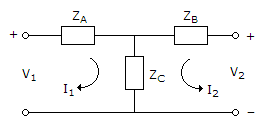Apply KVL to mesh 1

V1 = (ZA + ZC)I1 + ZCI2

Apply KVL to mesh 2

V2 = (ZB + ZC)I2 + ZCI1

Compare equation (i) and (iii)

ZA + ZC = 5j and ZA = 6j

ZA = - j

Compare equation (ii) and (iv)

ZB + ZC = 8j

ZB + j = 8j

ZB = 2j

ZA = - j ZB = 2j ZC = 6j.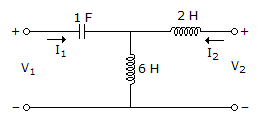#### Current Affairs 2022

Interview Questions and Answers